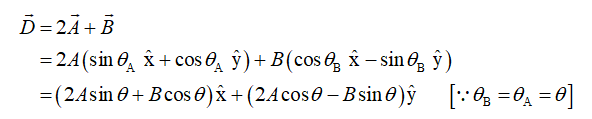# AAУУ2AL4 m160°20°-113mB.FIGURE P3.25FIGURE P3.2425. I FIGURE P3.25 shows vectors A and B. Find vector Č such theÁ +B + C = 0. Write your answer in component form.Ď = 2Á + B. Wite26. II FIGURE P3.26 shows vectors A and B. Find%3Iyour answer in component form. 25. I FIGURE P3.25 shows vectors A and B. Find vector Č200 *e of1-1FIGURE P3.25d theFIGURE P3.24remssuch thatÀ +B + C = Ō. Write your answer in component form26. I FIGURE P3.26 shows vectors À and B. Find Ď = 2Á + B. Writeyour answer in component form.islqxabuting2 m15°MA232IDAEX15°4mCC FIGURE P3.2627. || Find a vector that points in the same direction as the vector(î + î) and whose magnitude is 1.28. || While vacationing in the mountainsmorning

Question
10 views

#26

check_circle

The resultant vector,

Vector A is in first quadrant and B is in fourth quadrant.

Write the resultant in component form...

### Want to see the full answer?

See Solution

#### Want to see this answer and more?

Solutions are written by subject experts who are available 24/7. Questions are typically answered within 1 hour.*

See Solution
*Response times may vary by subject and question.
Tagged in

### Physics